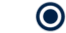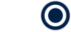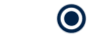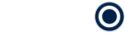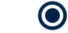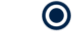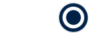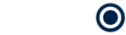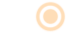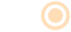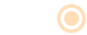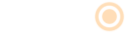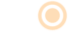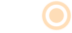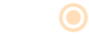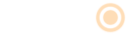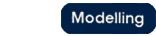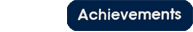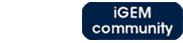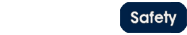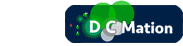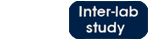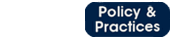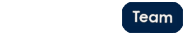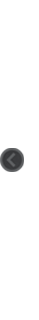# Team:Oxford/alternatives to microcompartments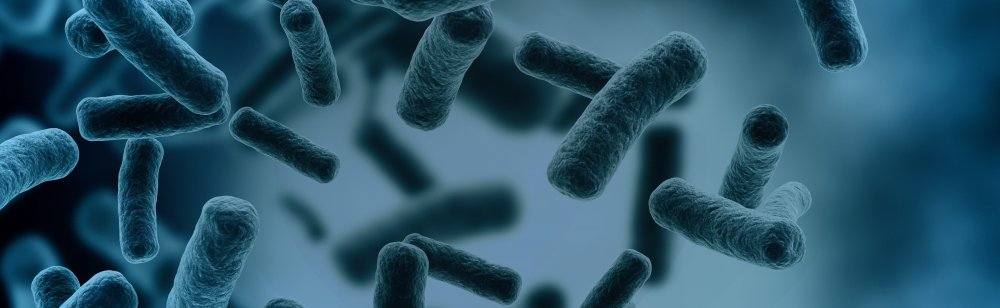Microcompartment Alternatives# Introduction: Helping the Melbourne team by creating models for their star peptide

The degradation pathway of DCM by DcmA produces a number of intermediates. Some of these, such as formaldehyde, are suspected to be toxic to our host bacteria above certain concentrations. Alongside using microcompartments for our project, we have also collaborated with UniMelb iGEM and considered attaching our different enzymes to the arms of a star peptide (https://2014.igem.org/Team:Melbourne) . Doing so would increase the likelihood that the reaction product of the first enzyme encounters the second enzyme in the pathway rapidly due to their proximity, therefore preventing the buildup of metabolic intermediates.

Our reaction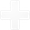Our reaction
Our reaction pathway is as follows:

Reaction 1: DCM + DcmA --> toxic intermediate (formaldehyde)

Reaction 2: toxic intermediate (formaldehyde) + FdhA --> neutral product (formate)

The Melbourne star peptide length can be varied in order to control the rate of reaction. Melbourne, however, were unsure of the form of the relationship between these length and rate and asked Oxford to develop a model of this. We hypothesized that, broadly speaking, the effect of increasing the length of the tether would result in a decrease in reaction rate due to two factors- the increased time taken to diffuse from one enzyme to the other and the decreased likelihood of colliding with an enzyme as the distance of diffusion increases.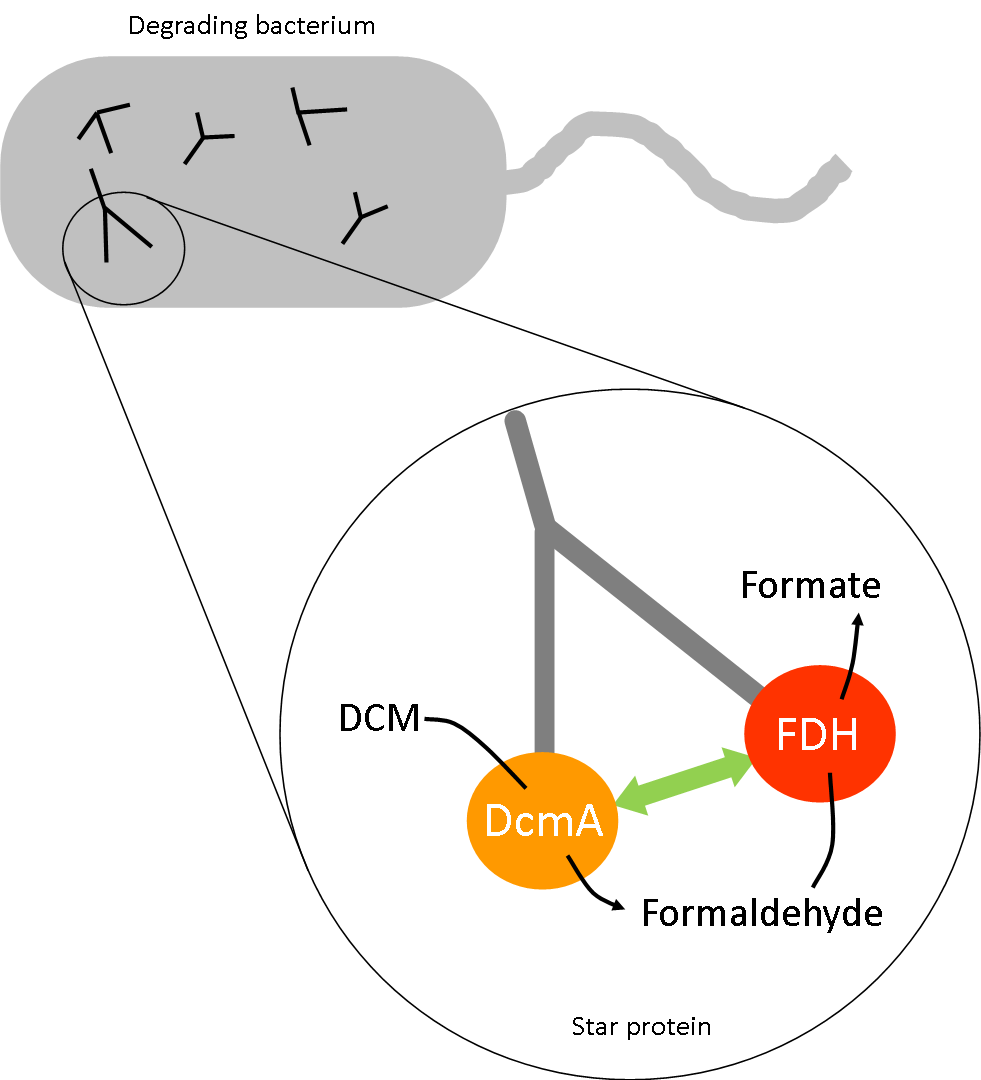# A visualization of the star peptide- the key variable is the distance between the active sites of the two key enzymes. We were asked to propose a relationship between this distance and reaction rate.

The star peptide modelThe model

# The star peptide- building the model

To isolate the effect of increased diffusion time, our simulation released 100 molecules from the same starting point and tracked their motion through stochastically driven diffusion. It then tracked the time required for 50 of these molecules to reach radii of varying length.

This is again based on another implicit assumption – that a collision between an enzyme and a substrate is a necessary but not sufficient condition for reaction to occur. Therefore, we have assumed that reaction rate is proportional, but not equal to, collision rate. As such, we cannot state the exact reaction rate and have normalized our data so that our highest data point takes a value 1 and subsequent reaction rates are expressed as fractions of this.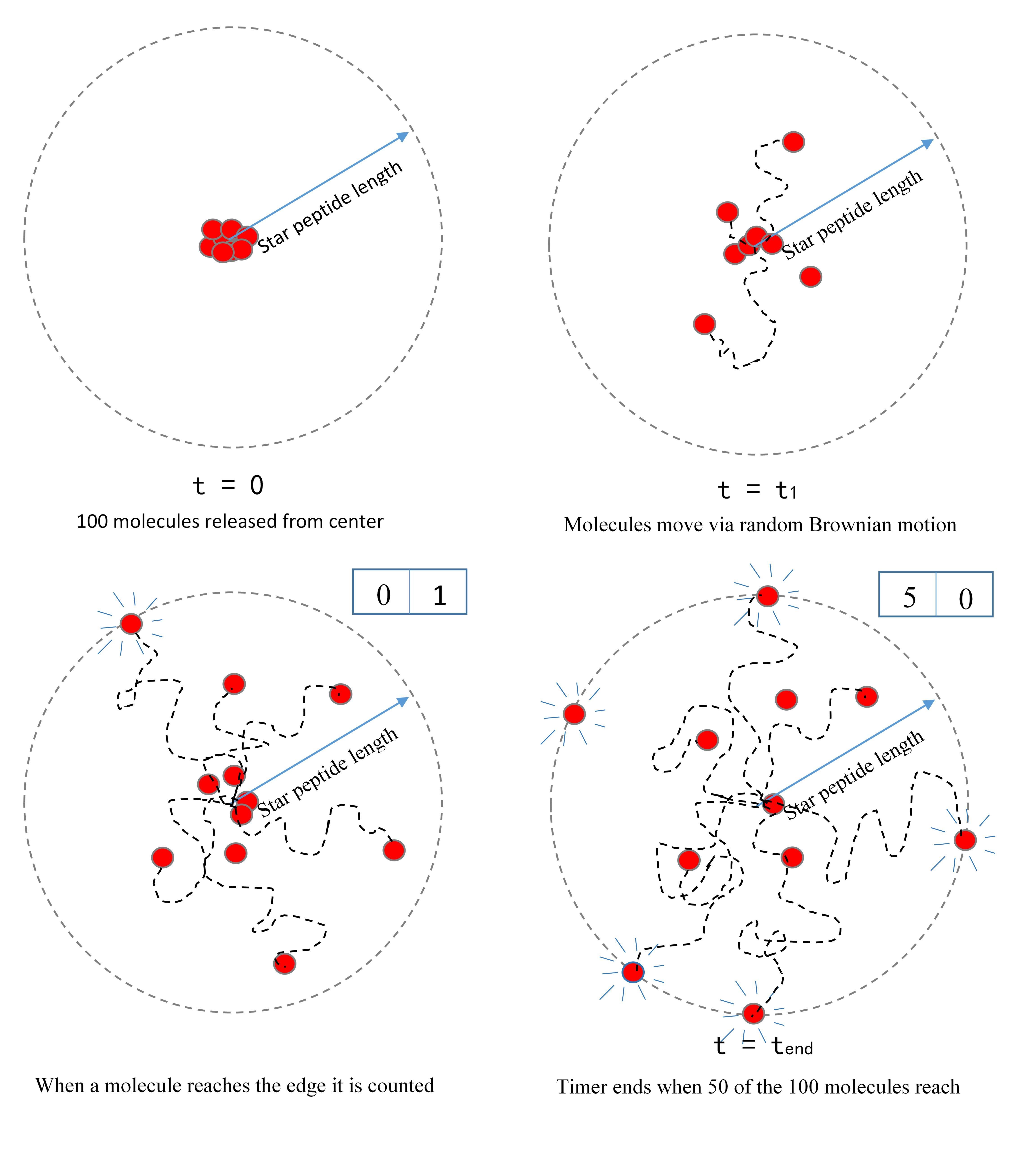Oxford iGEM 2014

# The star peptide- model results

The resulting relationship suggested that diffusion rate (defined as 1⁄t_end ) was proportional to 1⁄r^2 . In addition to this, we predict that the relative likelihood of a substrate colliding will an enzyme will decrease as the distance between the enzymes’ active sites increase. This will likely follow a 1⁄r^2 relationship if we consider the fact the likelihood of collision is inversely proportional to the fraction of the surface area of a sphere of the distance r that the enzyme occupies. Thus, we predict that the overall rate-distance relationship will take the form:

reaction rate ∝ 1⁄(peptide length)^4

Thus, the smaller the distance of separation, the higher we expect the rate of reaction to be. However, we must note that this model does not take into consideration stearic hindrances and instabilities that set in when the peptide is made too small. Furthermore, the model is only valid for a minimum radius which is defined as the sum of the two enzyme radii.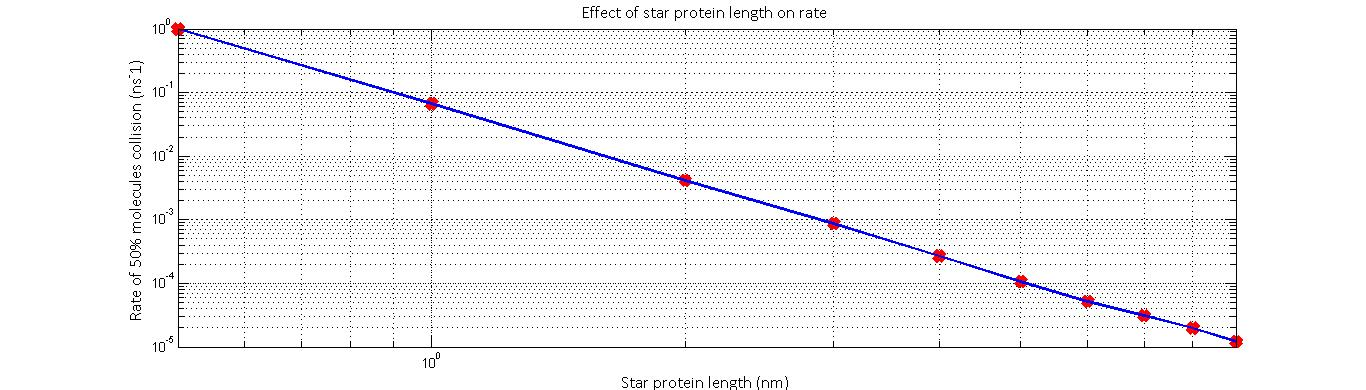# The normalized rate against star protein length results yielded by the model imply that reaction rate is proportional to peptide length^-4.

Calibrating the modelCalibrating the model

# Calibrating the stochastic diffusion model

To make our stochastic model realistic, we had to calibrate it against known diffusion distributions from Fick’s Law. The exact solution to Fick’s law suggests that the concentration spread will follow a Gaussian (Normal) distribution such that: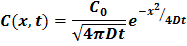Calculation of the diffusion constant of formaldehyde in cytoplasm proved difficult to find through literature. Thus, a theoretical value had to be substituted. From standard databases, the diffusion constant of formaldehyde in water is given as 2*(10^-5) cm^2 s^(-1).

Through comparison with this Gaussian, we could then calibrate our stochastic model to achieve maximum closeness of fit defined by: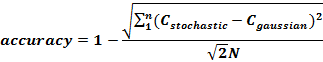The parameter being varied in the stochastic models is defined as ‘w0’- the relative likelihood of a molecule staying still within a certain time period, dt, rather than diffusing a pre-defined distance h. By varying w0 and calculating the median accuracy, we have identified the variable set [w0, h, dt] as [29, 0.25nm, 0.25ns] gives the closest resemblance to deterministic laws and was used in our model.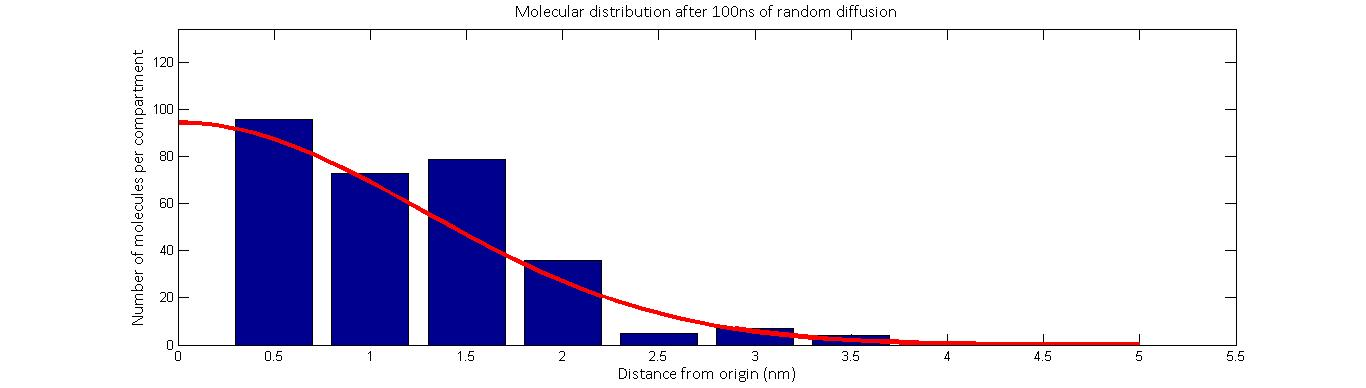# Plotted above is one simulation of the molecular distribution after 100 nanoseconds of random distribution. The red line represent the movement according to Fick’s law while the blue represents the calibrated stochastic diffusion model. As we see, there is good agreement between the two.

Further uses of the modelFurther uses of the model

# The star peptide- extending our results to other geometries

We should note that the only variable involved in this reaction is the distance between the active sites of the two enzymes involved in the reaction i.e. the point of production and the point of degradation. Combined with the fact that diffusion is a random process and equally likely in any direction, our results can be adapted to other geometries beyond a two enzyme tether. UniMelb iGEM informed as that they are able to synthesize two and four member systems and have developed a hypothetical method of synthesizing a six member system.

As long as we recognize which distance, D, we are concerned with, the model can be adapted to any number of systems and an amplification factor, A can be defined as: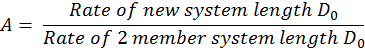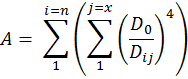• D_ij = distance between DcmA-i and FdhA-j
• D_0 = comparison distance
• x = number of FdhA molecules in system
• n = number of DcmA molecules in system

• Using this relationship, we can predict the relative efficiencies of each of these systems by calculating their amplification factor normalized over the number of molecules in the system. What our results suggest is that increasing the number of enzymes attached in the system will always increase the efficiency of the setup although there will naturally be limitations to the number of enzymes that can be attached in one system.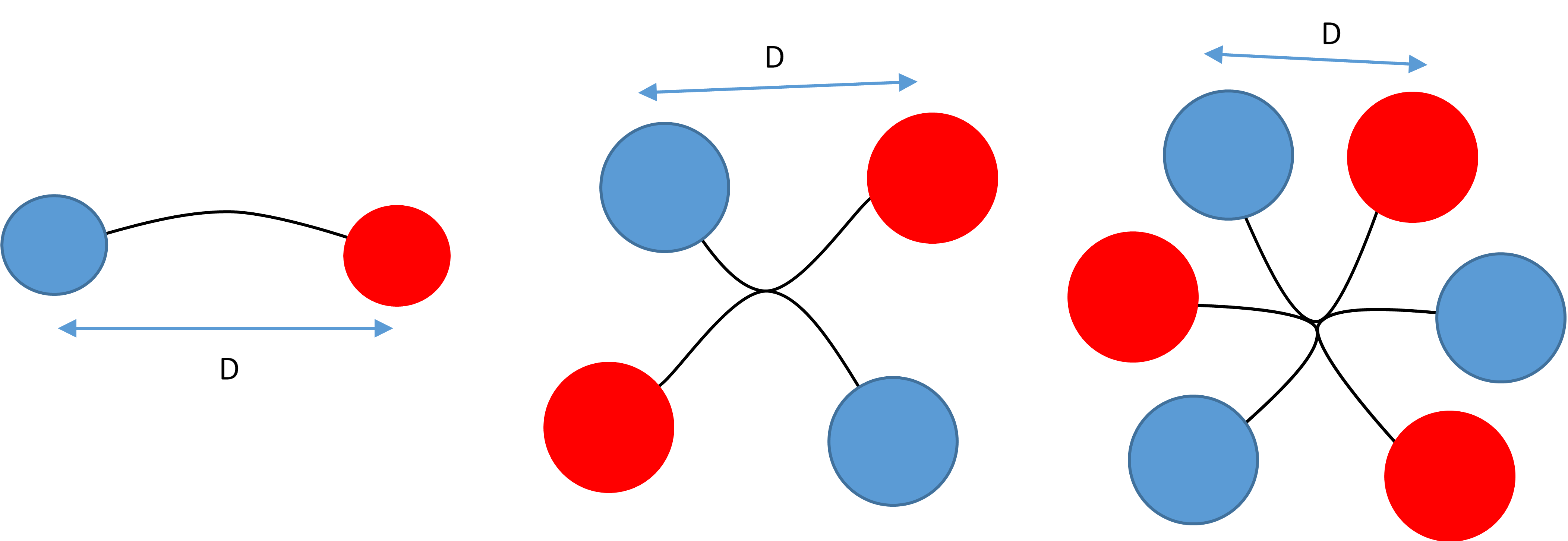# Visualisations of the two-member, four-member and six-member systems that UniMelb iGEM are able to synthesize. The current setups alternate the two enzyme species.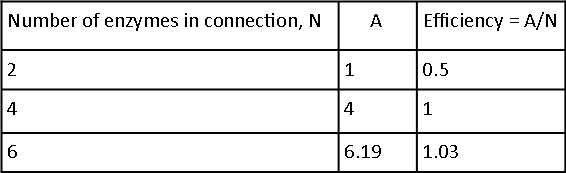# Calculating the amplification factor and relative efficiencies of each of the system geometries.